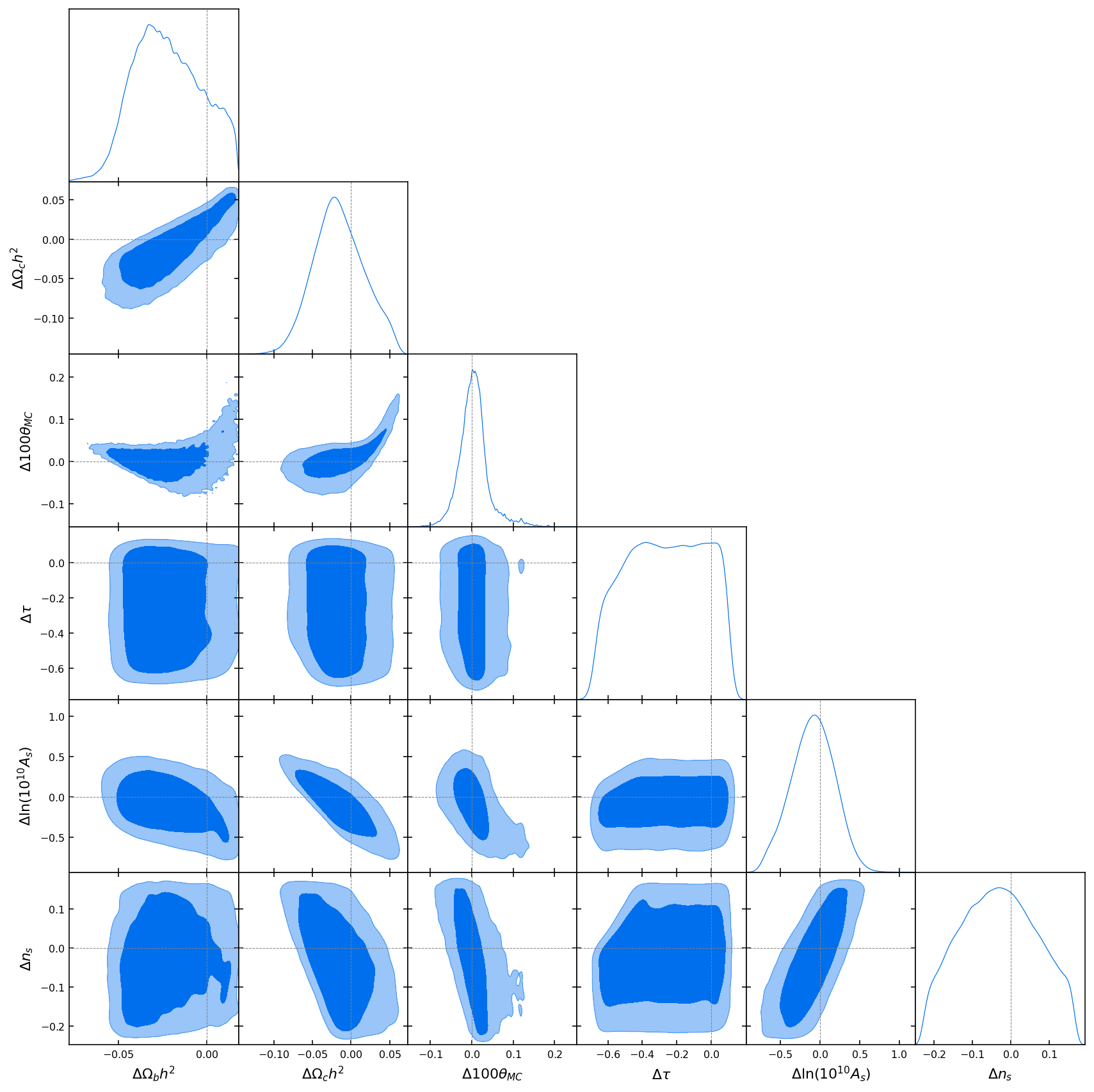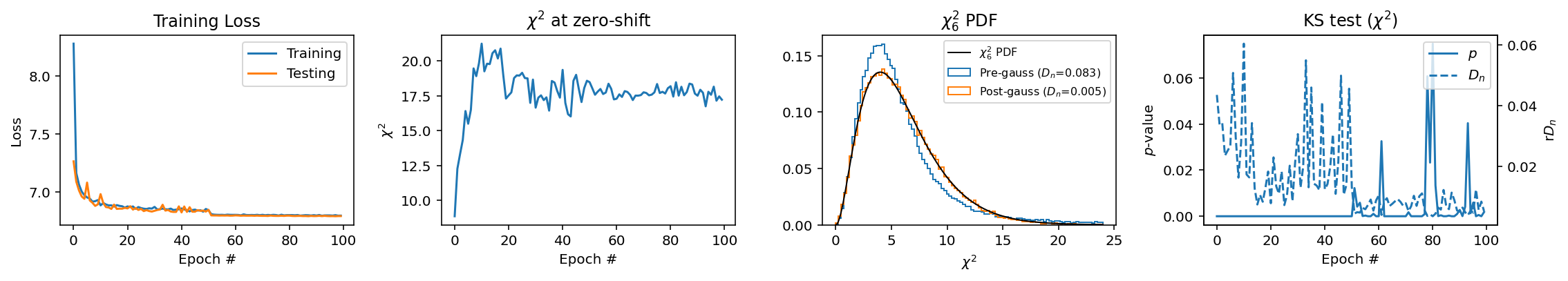# Non-Gaussian tension estimates¶

Cyrille Doux (cdoux@sas.upenn.edu), Marco Raveri (mraveri@sas.upenn.edu)

In this notebook we show how to compute the statistical significance of a parameter shift between two experiments, DES Y1 and Planck 18, with the two techniques discussed in Raveri and Doux (2021), arXiv:2105.03324.

In :
# Show plots inline, and load main getdist plot module and samples class
%matplotlib inline
%config InlineBackend.figure_format = 'retina'
# import libraries:
import sys, os
sys.path.insert(0,os.path.realpath(os.path.join(os.getcwd(),'../..')))
from getdist import plots, MCSamples
from getdist.gaussian_mixtures import GaussianND
import getdist
import scipy
import matplotlib.pyplot as plt
import IPython
from IPython.display import Markdown
import numpy as np
import seaborn as sns
# import the tensiometer tools that we need:
import tensiometer
from tensiometer import utilities
from tensiometer import gaussian_tension
from tensiometer import mcmc_tension


We start importing the chains and computing the parameter difference chain. We use the boost parameter to ensure that we enough samples for later.

In :
# load the samples (remove no burn in since the example chains have already been cleaned):
chains_dir = './../../test_chains/'
# the Planck 2018 TTTEEE chain:
# the DES Y1 3x2 chain:
# the difference chain:
diff_chain = mcmc_tension.parameter_diff_chain(chain_1, chain_2, boost=4)


Now we do a sanity check plot:

In :
param_names = diff_chain.getParamNames().getRunningNames()
g = plots.get_subplot_plotter()
g.triangle_plot([diff_chain], params=param_names, filled=True, markers={_p:0 for _p in param_names})Looks non-Gaussian uh? Let's see below how to cope with this!

# Normalizing flow estimate of parameter shifts:¶

The code provides a helper function, tensiometer.mcmc_tension.flow_parameter_shift(diff_chain), to create the model, train it and compute the shift significance. However, we will do these three steps manually to do some fine tuning and demonstrate some functionalities.

We first create the MAF model, with default architecture, but an initial learning rate of 0.01.

In :
diff_flow_callback = tensiometer.mcmc_tension.DiffFlowCallback(diff_chain, feedback=1, learning_rate=0.01)

Building training/test samples
- 1131315/125701 training/test samples and non-uniform weights.
Building MAF
- number of MAFs: 12
- activation: <function asinh at 0x7fa4c2fde290>
- hidden_units: [12, 12]
Building flow
- trainable parameters: 4752


We now train the model. To improve convergence, we started with a high learning rate and will use a Keras callback to decrease it when the validation loss is on a plateau.

In :
from tensorflow.keras.callbacks import ReduceLROnPlateau
callbacks = [ReduceLROnPlateau()]

batch_size = 8192
epochs = 100
steps_per_epoch = 128

diff_flow_callback.train(batch_size=batch_size, epochs=epochs, steps_per_epoch=steps_per_epoch, callbacks=callbacks)Out:
<tensorflow.python.keras.callbacks.History at 0x7fa4c00e4e50>
In :
exact_shift_P_1, exact_shift_low_1, exact_shift_hi_1 = diff_flow_callback.estimate_shift()
print(f'Shift probability considering all parameters = {exact_shift_P_1:.5f} +{exact_shift_hi_1-exact_shift_P_1:.5f} -{exact_shift_P_1-exact_shift_low_1:.5f}')
# turn the result to effective number of sigmas:
print(f'    n_sigma = {utilities.from_confidence_to_sigma(exact_shift_P_1):.3f}')

Shift probability considering all parameters = 0.99668 +0.00018 -0.00019
n_sigma = 2.936


Note that there is some variance in the result due to initialization. You can repeat the above calculation some times to evaluate the variance.

We can now plot the learned distribution. To do so we draw some samples from the learned distribution and then feed them to getdist plotting:

In :
N = 10000
X_sample = np.array(diff_flow_callback.dist_learned.sample(N))
diff_flow = MCSamples(samples=X_sample, names=param_names, label='Learned distribution')

In :
colors=['orange', 'dodgerblue', 'k']
g = plots.get_subplot_plotter()
g.settings.num_plot_contours = 2
gaussian_approx = tensiometer.gaussian_tension.gaussian_approximation(diff_chain)
g.triangle_plot([diff_chain, diff_flow, gaussian_approx], params=param_names,
filled=False, markers={_p:0 for _p in param_names},
colors=colors, diag1d_kwargs={'colors':colors})